# Selina Solutions Concise Maths Class 10 Chapter 11 Geometric Progression Exercise 11(D)

The important concept which is explained in this exercise is the sum of n terms of a G.P. Problems in this exercise are well created to give a strong knowledge of this concept. The Selina Solutions for Class 10 Maths is for students who want to improve their problem-solving skills as well as to clear doubts in the concept. The solutions are developed by experts at BYJU’S according to the latest ICSE marking patterns. Here, the answers to this exercise are present in the Selina Solutions Concise Maths Class 10 Chapter 11 Geometric Progression Exercise 11(D) PDF, which is available in the link below.

## Selina Solutions Concise Maths Class 10 Chapter 11 Geometric Progression Exercise 11(D) Download PDF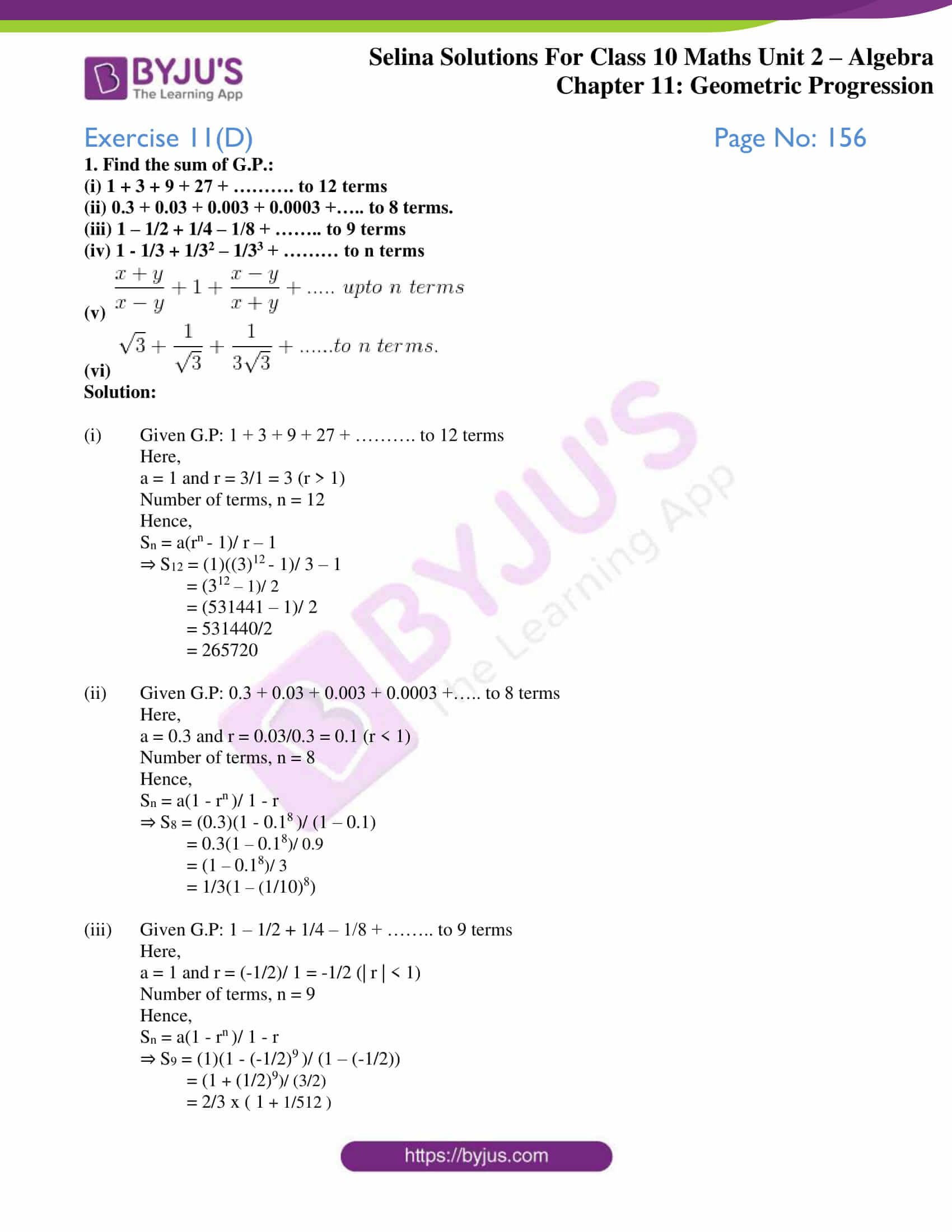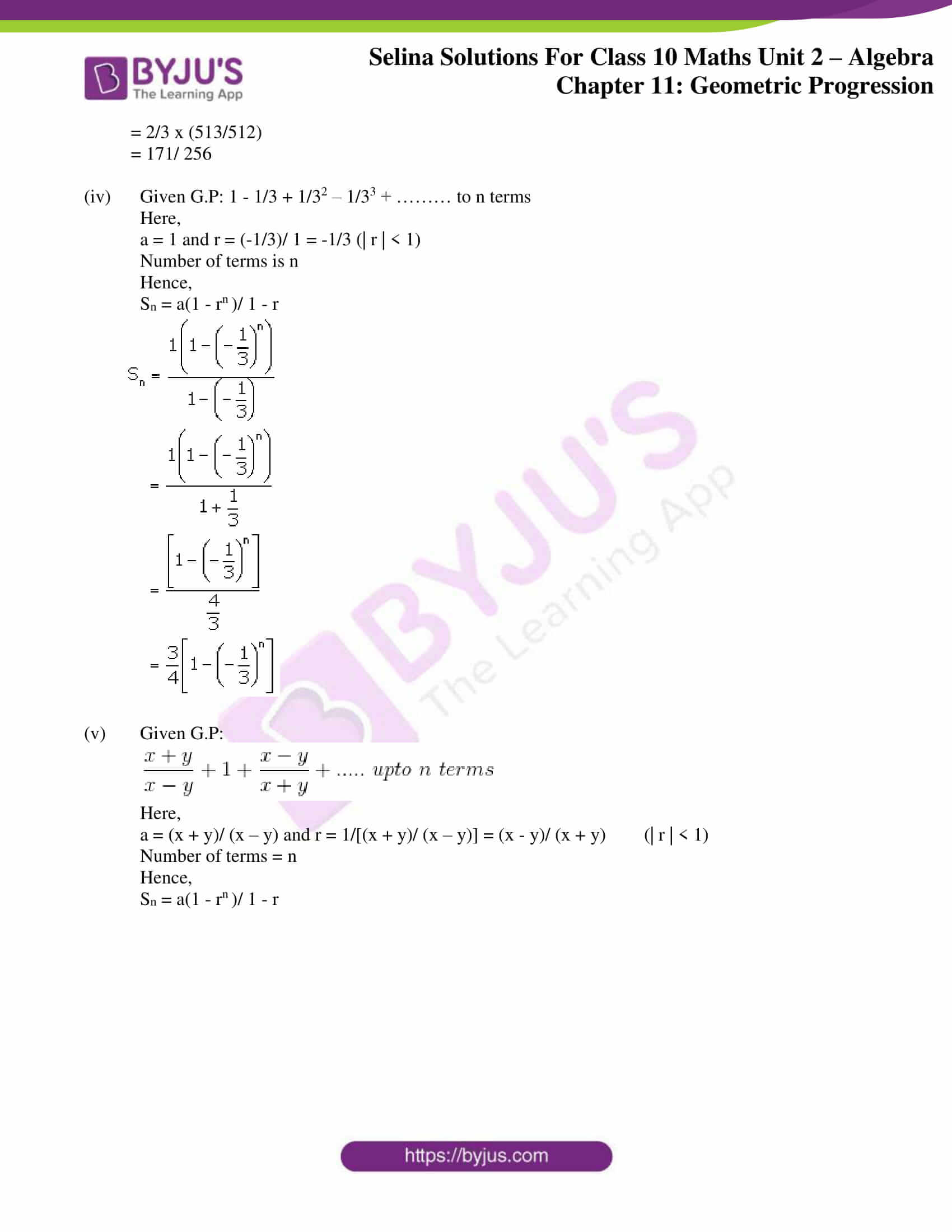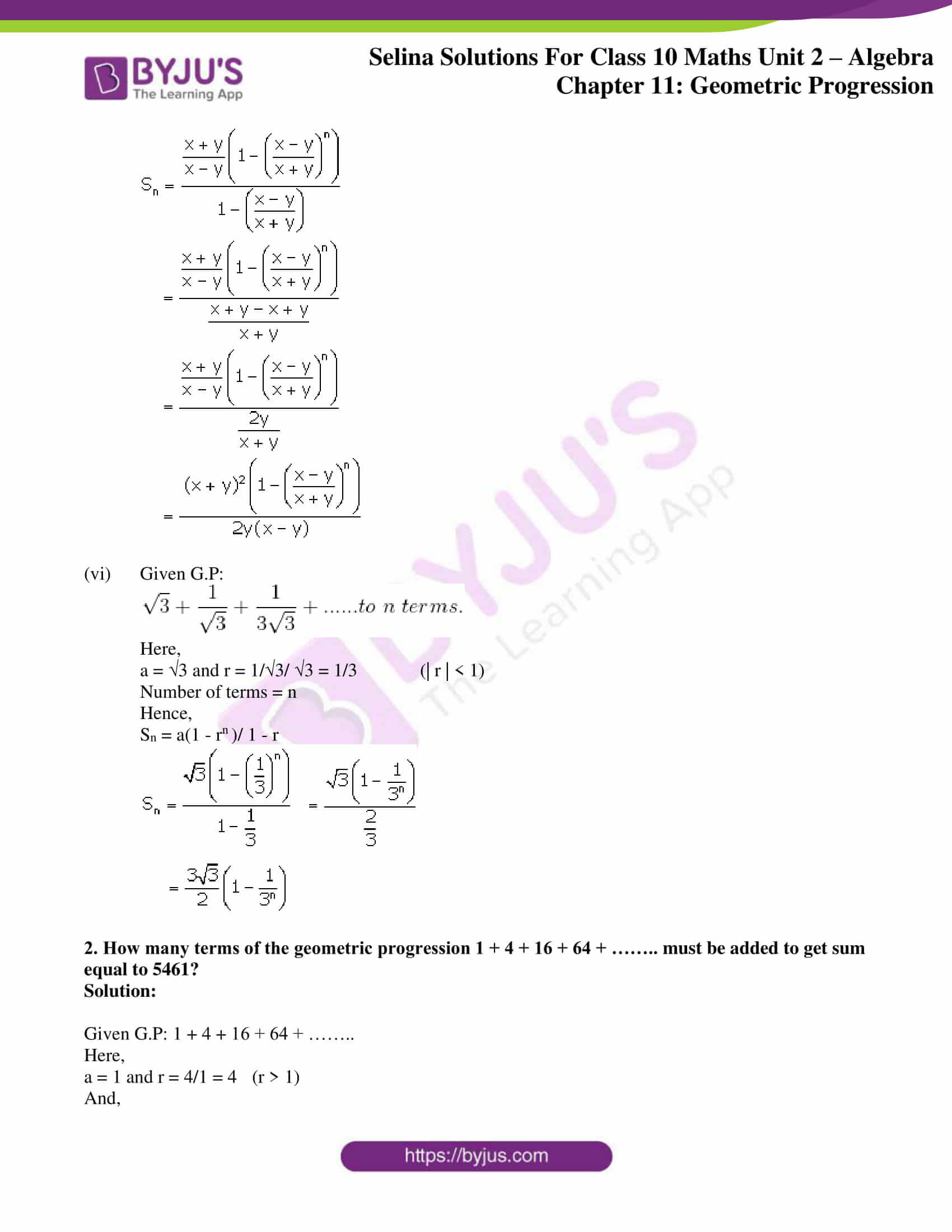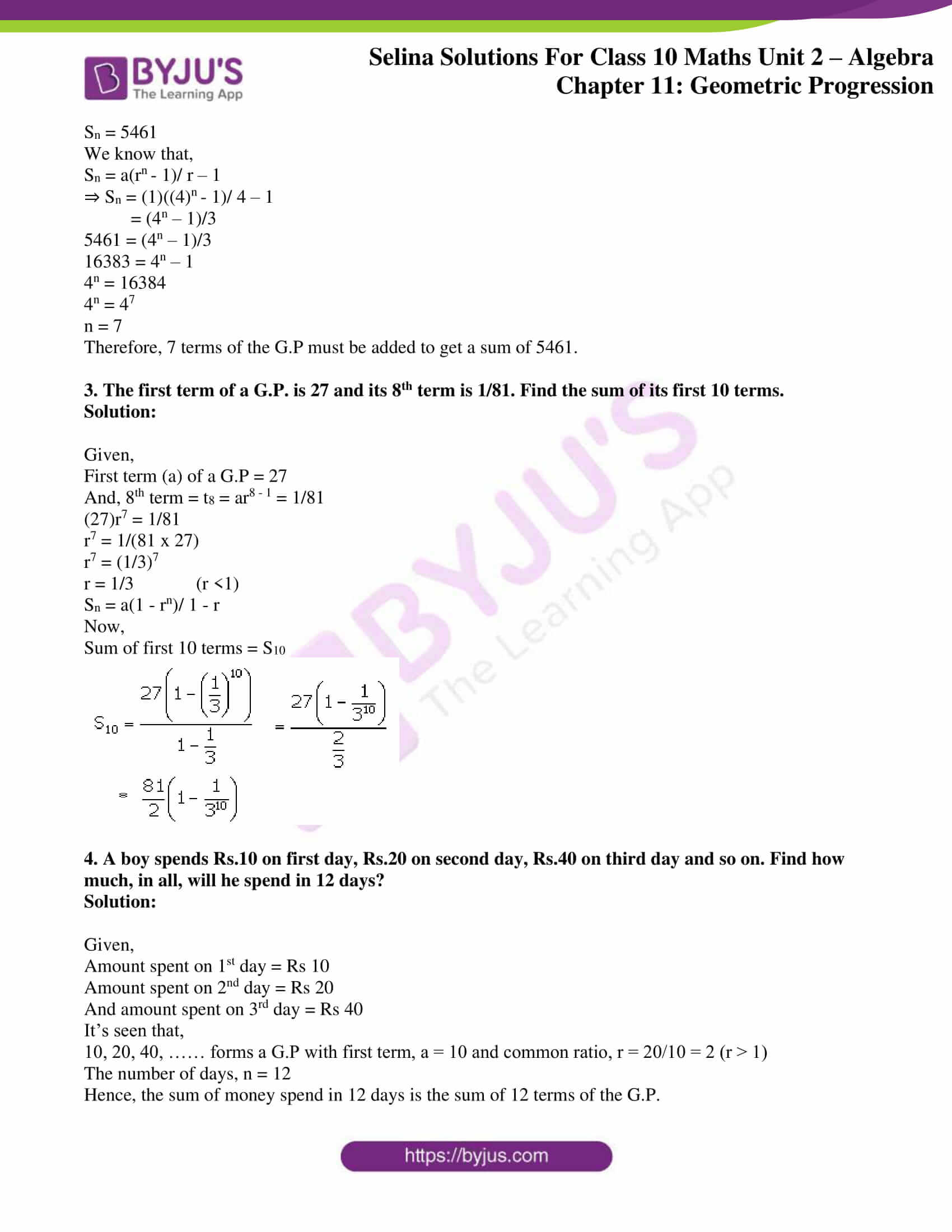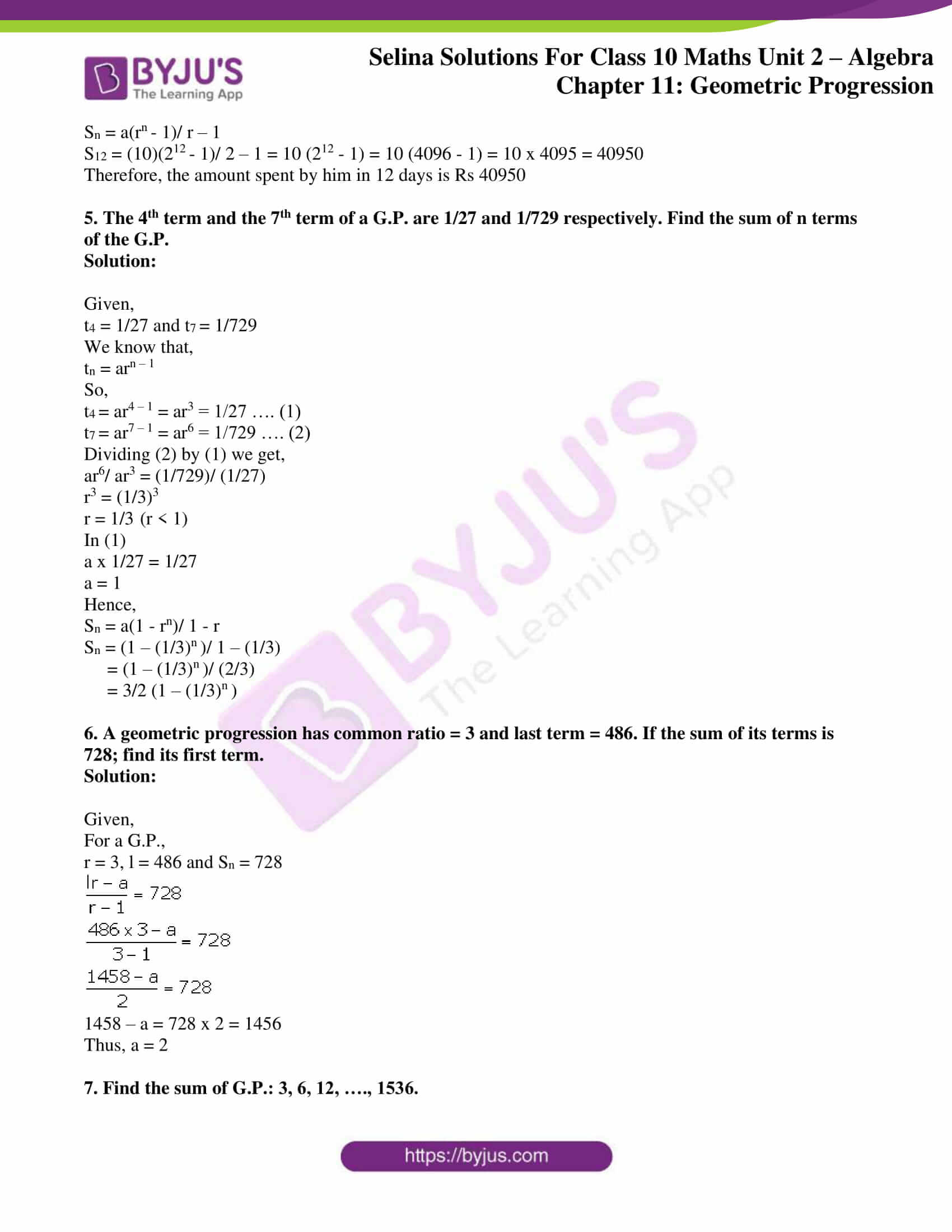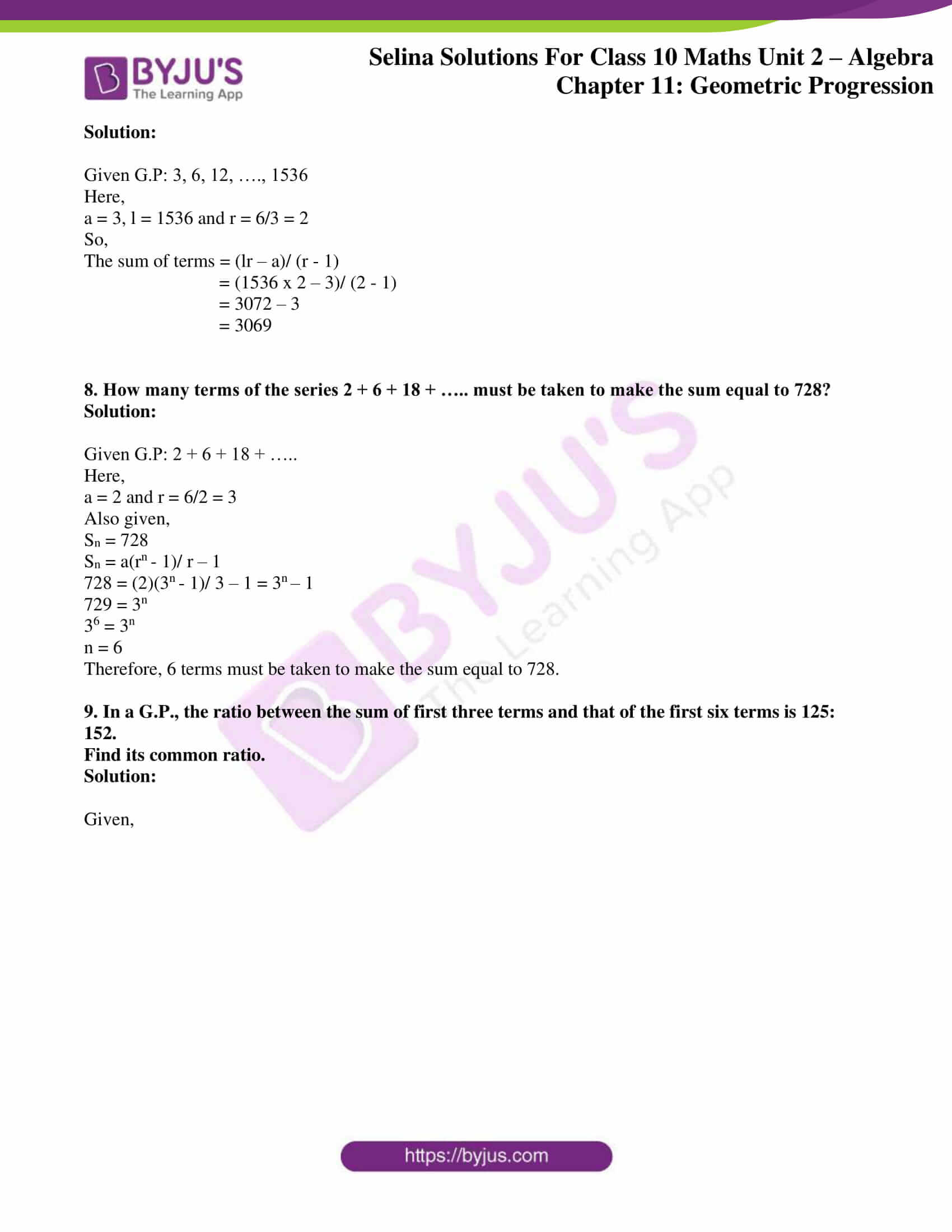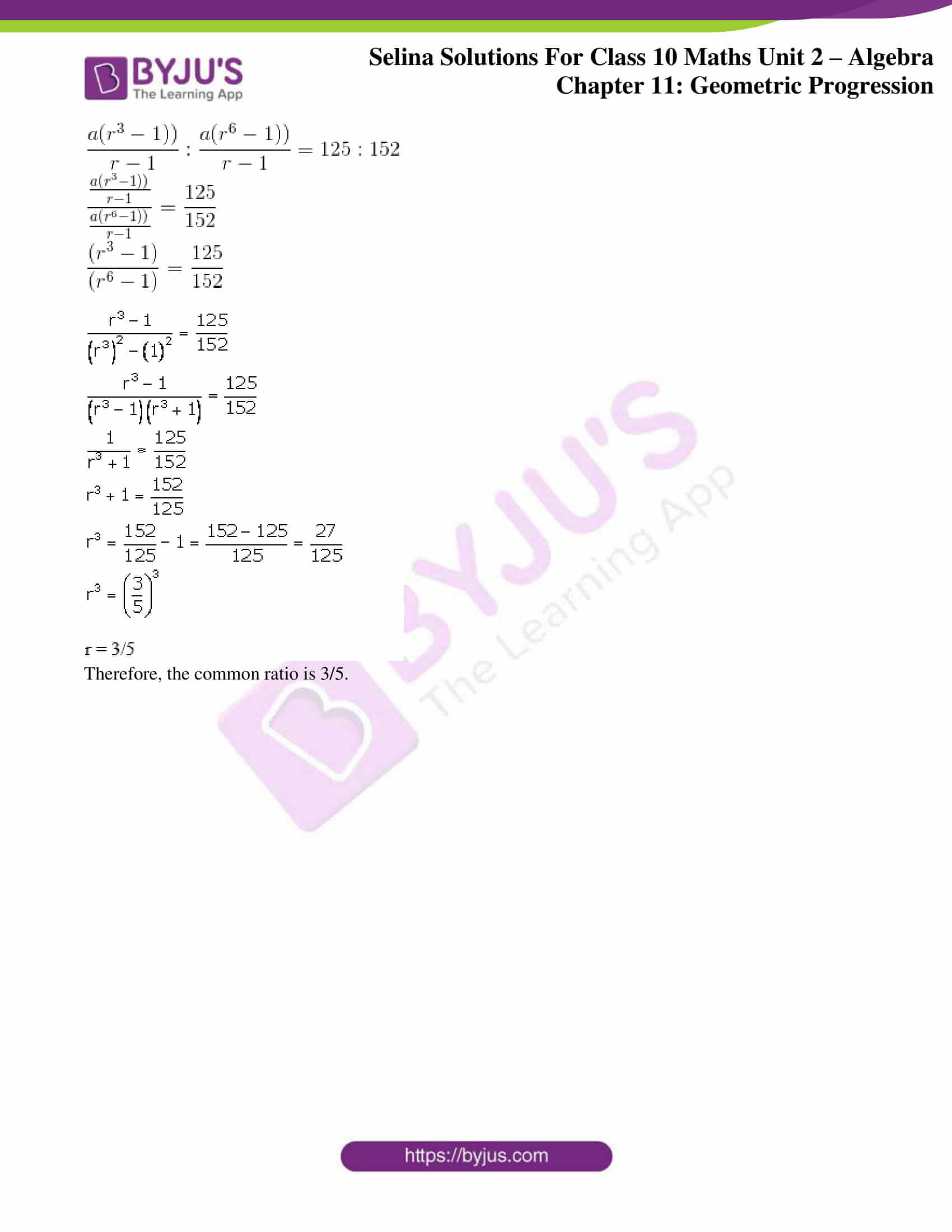### Access other exercises of Selina Solutions Concise Maths Class 10 Chapter 11 Geometric Progression

Exercise 11(A) Solutions

Exercise 11(B) Solutions

Exercise 11(C) Solutions

### Access Selina Solutions Concise Maths Class 10 Chapter 11 Geometric Progression Exercise 11(D)

#### Exercise 11(D) Page No: 156

1. Find the sum of G.P.:

(i) 1 + 3 + 9 + 27 + ………. to 12 terms

(ii) 0.3 + 0.03 + 0.003 + 0.0003 +….. to 8 terms.

(iii) 1 – 1/2 + 1/4 – 1/8 + …….. to 9 terms

(iv) 1 – 1/3 + 1/32 – 1/33 + ……… to n terms

(v)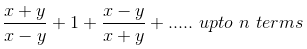(vi)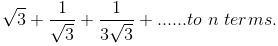Solution:

(i) Given G.P: 1 + 3 + 9 + 27 + ………. to 12 terms

Here,

a = 1 and r = 3/1 = 3 (r > 1)

Number of terms, n = 12

Hence,

Sn = a(rn – 1)/ r – 1

⇒ S12 = (1)((3)12 – 1)/ 3 – 1

= (312 – 1)/ 2

= (531441 – 1)/ 2

= 531440/2

= 265720

(ii) Given G.P: 0.3 + 0.03 + 0.003 + 0.0003 +….. to 8 terms

Here,

a = 0.3 and r = 0.03/0.3 = 0.1 (r < 1)

Number of terms, n = 8

Hence,

Sn = a(1 – rn )/ 1 – r

⇒ S8 = (0.3)(1 – 0.18 )/ (1 – 0.1)

= 0.3(1 – 0.18)/ 0.9

= (1 – 0.18)/ 3

= 1/3(1 – (1/10)8)

(iii) Given G.P: 1 – 1/2 + 1/4 – 1/8 + …….. to 9 terms

Here,

a = 1 and r = (-1/2)/ 1 = -1/2 (| r | < 1)

Number of terms, n = 9

Hence,

Sn = a(1 – rn )/ 1 – r

⇒ S9 = (1)(1 – (-1/2)9 )/ (1 – (-1/2))

= (1 + (1/2)9)/ (3/2)

= 2/3 x ( 1 + 1/512 )

= 2/3 x (513/512)

= 171/ 256

(iv) Given G.P: 1 – 1/3 + 1/32 – 1/33 + ……… to n terms

Here,

a = 1 and r = (-1/3)/ 1 = -1/3 (| r | < 1)

Number of terms is n

Hence,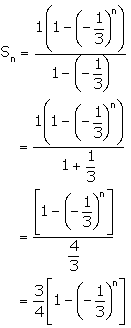Sn = a(1 – rn )/ 1 – r

(v) Given G.P:Here,

a = (x + y)/ (x – y) and r = 1/[(x + y)/ (x – y)] = (x – y)/ (x + y) (| r | < 1)

Number of terms = n

Hence,

Sn = a(1 – rn )/ 1 – r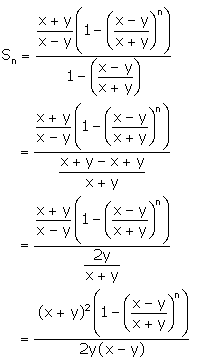(vi) Given G.P:Here,

a = √3 and r = 1/√3/ √3 = 1/3 (| r | < 1)

Number of terms = n

Hence,

Sn = a(1 – rn )/ 1 – r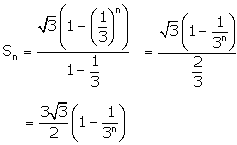2. How many terms of the geometric progression 1 + 4 + 16 + 64 + …….. must be added to get sum equal to 5461?

Solution:

Given G.P: 1 + 4 + 16 + 64 + ……..

Here,

a = 1 and r = 4/1 = 4 (r > 1)

And,

Sn = 5461

We know that,

Sn = a(rn – 1)/ r – 1

⇒ Sn = (1)((4)n – 1)/ 4 – 1

= (4n – 1)/3

5461 = (4n – 1)/3

16383 = 4n – 1

4n = 16384

4n = 47

n = 7

Therefore, 7 terms of the G.P must be added to get a sum of 5461.

3. The first term of a G.P. is 27 and its 8th term is 1/81. Find the sum of its first 10 terms.

Solution:

Given,

First term (a) of a G.P = 27

And, 8th term = t8 = ar8 – 1 = 1/81

(27)r7 = 1/81

r7 = 1/(81 x 27)

r7 = (1/3)7

r = 1/3 (r <1)

Sn = a(1 – rn)/ 1 – r

Now,

Sum of first 10 terms = S10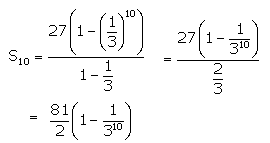4. A boy spends Rs.10 on first day, Rs.20 on second day, Rs.40 on third day and so on. Find how much, in all, will he spend in 12 days?

Solution:

Given,

Amount spent on 1st day = Rs 10

Amount spent on 2nd day = Rs 20

And amount spent on 3rd day = Rs 40

It’s seen that,

10, 20, 40, …… forms a G.P with first term, a = 10 and common ratio, r = 20/10 = 2 (r > 1)

The number of days, n = 12

Hence, the sum of money spend in 12 days is the sum of 12 terms of the G.P.

Sn = a(rn – 1)/ r – 1

S12 = (10)(212 – 1)/ 2 – 1 = 10 (212 – 1) = 10 (4096 – 1) = 10 x 4095 = 40950

Therefore, the amount spent by him in 12 days is Rs 40950

5. The 4th term and the 7th term of a G.P. are 1/27 and 1/729 respectively. Find the sum of n terms of the G.P.

Solution:

Given,

t4 = 1/27 and t7 = 1/729

We know that,

tn = arn – 1

So,

t4 = ar4 – 1 = ar3 = 1/27 …. (1)

t7 = ar7 – 1 = ar6 = 1/729 …. (2)

Dividing (2) by (1) we get,

ar6/ ar3 = (1/729)/ (1/27)

r3 = (1/3)3

r = 1/3 (r < 1)

In (1)

a x 1/27 = 1/27

a = 1

Hence,

Sn = a(1 – rn)/ 1 – r

Sn = (1 – (1/3)n )/ 1 – (1/3)

= (1 – (1/3)n )/ (2/3)

= 3/2 (1 – (1/3)n )

6. A geometric progression has common ratio = 3 and last term = 486. If the sum of its terms is 728; find its first term.

Solution:

Given,

For a G.P.,

r = 3, l = 486 and Sn = 728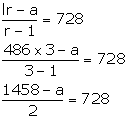1458 – a = 728 x 2 = 1456

Thus, a = 2

7. Find the sum of G.P.: 3, 6, 12, …., 1536.

Solution:

Given G.P: 3, 6, 12, …., 1536

Here,

a = 3, l = 1536 and r = 6/3 = 2

So,

The sum of terms = (lr – a)/ (r – 1)

= (1536 x 2 – 3)/ (2 – 1)

= 3072 – 3

= 3069

8. How many terms of the series 2 + 6 + 18 + ….. must be taken to make the sum equal to 728?

Solution:

Given G.P: 2 + 6 + 18 + …..

Here,

a = 2 and r = 6/2 = 3

Also given,

Sn = 728

Sn = a(rn – 1)/ r – 1

728 = (2)(3n – 1)/ 3 – 1 = 3n – 1

729 = 3n

36 = 3n

n = 6

Therefore, 6 terms must be taken to make the sum equal to 728.

9. In a G.P., the ratio between the sum of first three terms and that of the first six terms is 125: 152.

Find its common ratio.

Solution:

Given,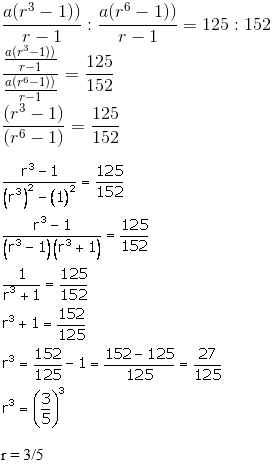Therefore, the common ratio is 3/5.